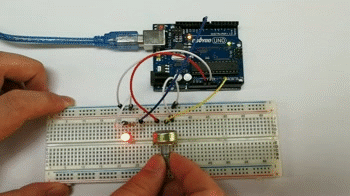# Introduction

In this lesson, we will use a potentiometer attached to analog pin A0 and an LED attached to digital pin 9. We use the input from the analog pin to adjust the timing delay of a blinking LED.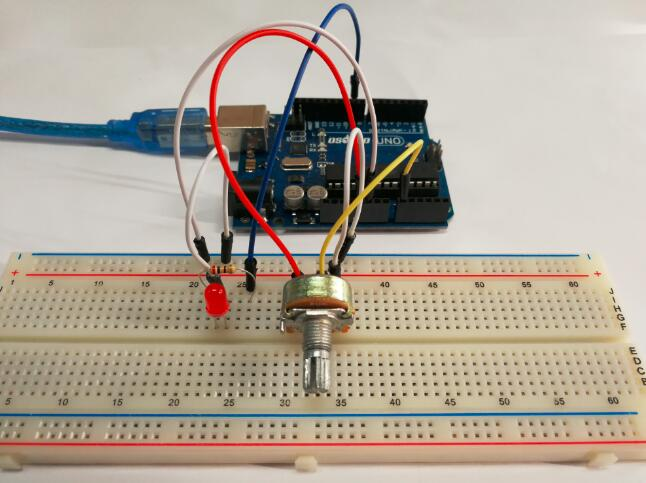# Preparations

## Hardware

• Osoyoo UNO Board (Fully compatible with Arduino UNO rev.3) x 1
• Potentiometer(10k) x 1
• 200 ohm resistor x 1
• LED x 1
• M/M jumpers
• USB Cable x 1
• PC x 1

## Software

Arduino IDE (version 1.6.4+)A potentiometer is a simple knob that provides a variable resistance, which we can read into the Arduino board as an analog value.In this example, that value controls the rate at which an LED blinks.

So what’s the difference between an analog value and a digital one? Simply put, digital means on/off, high/low level with just two states, i.e. either 0 or 1. But the data state of analog signals is linear, for example, from 1 to 1000; the signal value changes over time instead of indicating an exact number. Analog signals include those of light intensity, humidity, temperature, and so on.

In this lesson, a potentiometer, or pot, is used to change the current in the circuit so the blink rate of the LED will change accordingly. And since the pot is an analog device, the current change is smooth, thus the blink rate will gradually get faster or slower instead of going through an obvious stepwise process.

What we mean by PWM here is the digitalization of analog signals, which is a process of approaching analog signals. Since the potentiometer inputs analog signals, it should be connected to analog ports, i.e. A0-A5, instead of digital ports.

# Connection

Build the circuit as below: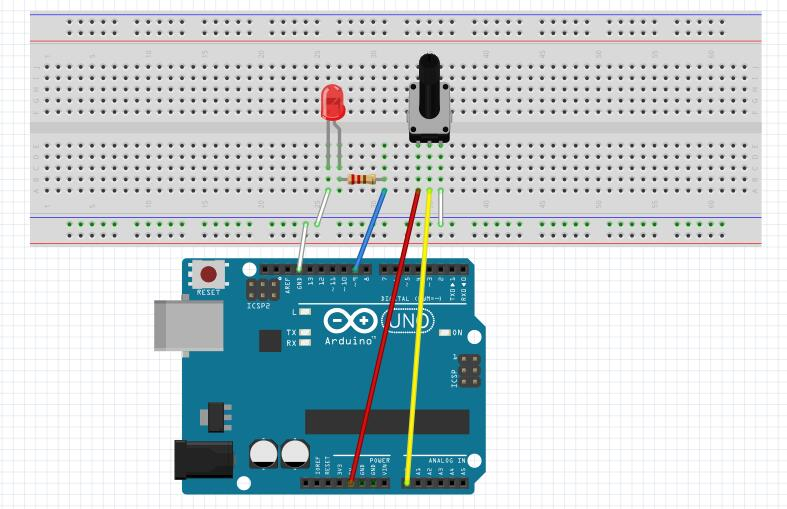We connect three wires to the Arduino board. The first goes to ground from one of the outer pins of the potentiometer. The second goes from 5 volts to the other outer pin of the potentiometer. The third goes from analog input 0 to the middle pin of the potentiometer.

After above operations are completed, connect the Arduino board to your computer using the USB cable. The green power LED (labelled PWR) should go on.

## Code Program

```int potPin = A0;    // select the input pin for the potentiometer
int ledPin = 9;   // select the pin for the LED
int val = 0;       // variable to store the value coming from the sensor

void setup() {
pinMode(ledPin, OUTPUT);  // declare the ledPin as an OUTPUT
}

void loop() {
digitalWrite(ledPin, HIGH);  // turn the ledPin on
delay(val);                  // stop the program for some time
digitalWrite(ledPin, LOW);   // turn the ledPin off
delay(val);                  // stop the program for some time
}
```

By turning the shaft of the potentiometer, we change the amount of resistence on either side of the wiper which is connected to the center pin of the potentiometer. This changes the relative “closeness” of that pin to 5 volts and ground, giving us a different analog input. When the shaft is turned all the way in one direction, there are 0 volts going to the pin, and we read 0. When the shaft is turned all the way in the other direction, there are 5 volts going to the pin and we read 1023. In between, analogRead() returns a number between 0 and 1023 that is proportional to the amount of voltage being applied to the pin.

Open the Arduino IDE and select corresponding board type and port type for your Arduino board.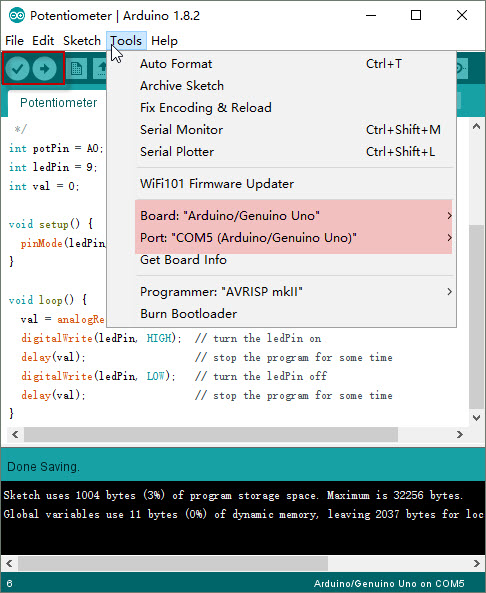After compile this sketch, simply click the “Upload” button in the environment. Wait a few seconds – you should see the RX and TX leds on the board flashing. If the upload is successful, the message “Done uploading.” will appear in the status bar.

# Running Result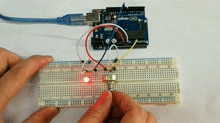# Extended experiment

In the previous course, you have learned how to control an LED by PWM programming, which is interesting though sounds slightly abstract. In this part, you will learn how to change the luminance of an LED by potentiometer.

The circuit of this part is same as above.You can get the sketch from this link or copy below code to your new Arduino IDE window and upload it to your arduino board. Don’t forget to choose the corresponding board and port for you project!

```const int analogPin = A0;//the analog input pin attach to
const int ledPin = 9;//the led attach to
int inputValue = 0;//variable to store the value coming from sensor
int outputValue = 0;//variable to store the output value

void setup()
{
}

void loop()
{